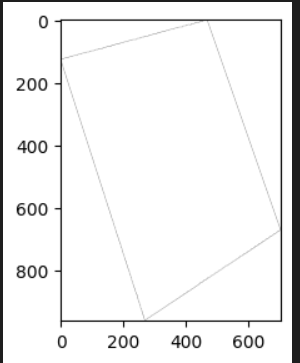# Visualize pleiades image using process API

Hi,

I have purchased a pleiades image. I can visualize it using EO browser, however , visualizing it with python doesn’t work.
I have followed this tutorial in order to visualize the image.

this is what I did:

``````# Initialize SentinelHubBYOC class

byoc = SentinelHubBYOC(config=config)

#airbus plaiedas

my_collection = byoc.get_collection('THIS_IS_SECRET')

print(f"name: {my_collection['name']}")

print(f"id: {my_collection['id']}")

#get list of tiles inside the collection
tiles = list(byoc.iter_tiles(my_collection))

#convert it to ByocTile dataclass

tile = ByocTile.from_dict(next(byoc.iter_tiles(my_collection)))

#visualized tiles

tile_iterator = byoc.iter_tiles(my_collection)

tiles_for_visualized = []

#should be for in in range(#number of images)

for i in :

tiles_for_visualized.append(ByocTile.from_dict(next(tile_iterator)))

tiles_gdf = gpd.GeoDataFrame(

tiles_for_visualized,

geometry=[t.cover_geometry.transform(CRS.WGS84).geometry for t in tiles_for_visualized],

crs="epsg:4326",)

data_collection = DataCollection.define_byoc(my_collection["id"])

#######THIS PART DOESN'T WORK#########
tile_time = dt.datetime.fromisoformat(tiles["sensingTime"])
``````

ValueError: Invalid isoformat string: ‘2022-09-25T19:08:28.500Z’

I have tried to skip this by manually insert the date ‘2022-09-25’ and send the request but that yield an empty image:

``````bbox_size,bbox,bbox_coords_wgs84=get_bbox_from_shape(tiles_gdf,0.5)
evalscript = """

//VERSION=3

function setup() {

return {

input: ["B3","B2","B1", "B0"],

output: { bands: 4 },

};

}

function evaluatePixel(sample) {

return [sample.B0, sample.B1,sample.B2,sample.B3];

}

"""

request = SentinelHubRequest(

evalscript=evalscript,

input_data=[SentinelHubRequest.input_data(data_collection=data_collection, time_interval='2022-09-25')],

responses=[SentinelHubRequest.output_response("default", MimeType.TIFF)],

bbox=bbox,

size=bbox_size,

config=config,

)
``````

That gives me an image with the borders of the polygon of the image but no values:My questions are:

1. I think there is problem with the tutorial with the date format shown there, seems like it works with the format I inserted manually?
2. How can I visualize my image? what is wrong with my script?

Thanks in advanceHi Reut,

1. I think the problem with your date here in the request is that you need to specify a range like this:
``````time_interval=('2017-09-01', '2017-09-28'),
``````
1. To visualise Pleaides bands correctly you should use `sample.B1 / 10000` for each band to convert from DN to reflectance. This is due to the units used.

In addition, you can visit this page for some examples for Pleaides in our documentation. To see them in Python you can parse the curl request into Request Builder then change the language type to Python.

1 Like

Hi Wiliam!

However, for the date issue, I still get errors.
I have tried to change it as you suggested but it didn’t work:

`````` input_data=[SentinelHubRequest.input_data(data_collection=data_collection, time_interval=('2021-09-26','2021-09-29')]
``````

However, it does work with the original line (I put here sample date):

``````input_data=[SentinelHubRequest.input_data(data_collection=data_collection, time_interval='2021-09-27')]
``````

However, the part with the date that I’m more confused about is this:

``````tile_time = dt.datetime.fromisoformat(tiles["sensingTime"])
>>>
ValueError                                Traceback (most recent call last)
Cell In, line 1
----> 1 tile_time = dt.datetime.fromisoformat(tiles["sensingTime"])

ValueError: Invalid isoformat string: '2021-09-27T19:08:28.500Z'
``````

Hi Reut, so there is a bit of an issue in the tutorial you followed. Replacing the problematic line with

`tile_time = dt.datetime.fromisoformat(tiles["sensingTime"].replace("Z", "+00:00"))`

should make it work. Hope that fixes the issue!

1 Like

I have also found solution that worked, sharing it here
``````str(gdf['sensing_time'].values).split('T')